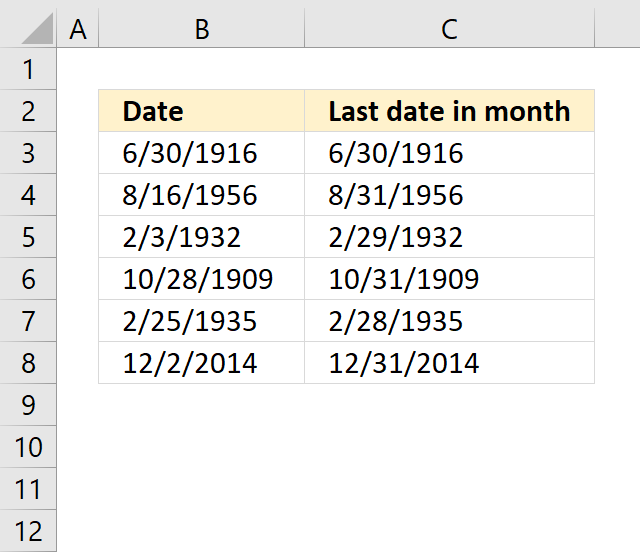Author: Oscar Cronquist Article last updated on November 16, 2018The formula in cell C3 calculates the last date for the given month and year in cell B3.

=DATE(YEAR(B3), MONTH(B3)+1, 1)-1

### Explaining formula in cell C3

The DATE function returns an Excel date based on a year, month and day number. DATE(year, month, day) but first we need to extract the argument numbers needed.

The YEAR function calculates the year of an Excel date value. YEAR(B3) becomes YEAR(6026) and returns 1916.

The MONTH function returns the number of the month of an Excel date value. However, we also add 1 to that number. This move takes us to the next month.

MONTH(B3)+1 becomes MONTH(6026)+1 becomes 6 + 1 and returns 7.

The third argument will always be 1. This makes sure that the DATE function always returns the first date of the next month. The DATE function now looks like this:

DATE(1906, 7, 1) and returns 7/1/1906.

But wait, we needed the last date in the previous month? Yes, but subtract the Excel date with 1 and you get the last date of the previous month. This takes care of weird things like leap years that might cause trouble to the calculations.

7/1/1906 -1 is 6/30/1906.